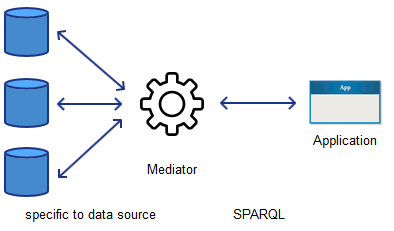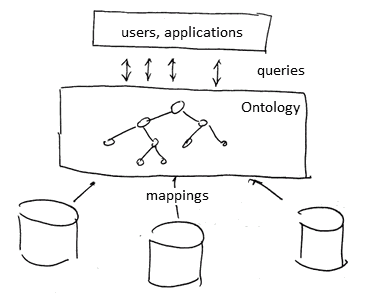# ML Wiki

## Mediator

This is an approach to Data Integration (opposite to Data Warehousing)

• data remains in the data sources (so it's sometimes called "virtual data integration")
• also better if you want to access "fresh" data
• but way harder to implement - need to transform data during the query time
•### Architecture

Global Schema

• start by designing a global (mediated) schema - an unique entry point for all the queries
• need to have semantic mappings between the mediated schema and data sources

Querying:

• user queries the global schema
• based on the mappings, queries are converted to local queries for the data sources
• all queries are executed
• then the results are combined (e.g. using some Ontologies - which is why this approach is useful for OBDA)

## Semantic Mapping

Let $S_1, ..., S_n$ be local schemas

• assume that each $S_i$ has only one relation, also denoted $S_i$
• these $S_1, ..., S_n$ are local relations

Global schema $G$

• consists of global relations $G_1, ..., G_m$

Goal of Semantic Mappings:

• specify mappings between $\{ S_i \}$ and $\{ G_i \}$ - relationships between local and global schemas
• examples of such relationships:
• $G_1 \equiv S_1$ - equality
• $G_2 \equiv S_1 \cup S_2$
• $G_3 \equiv S_1 \Join S_3$
• so global $G_j$ can be seen as views of local relationships (example of GAV Mediation)

But better to use containment instead of equality

• to be able to express the usage of multiple sources
• example (GAV Mediation)
• $G_3 \supseteq S_1 \Join S_3$
• $G_3 \supseteq \sigma_{A = \text{yes}} ( S_4 )$
• example (LAV Mediation)
• $S_4 \subseteq G_1 \Join G_3$

Notation

• $v(S_1, ..., S_n)$ - local view (a view/query built on local schemas)
• $v(G_1, ..., G_m)$ - global view (on global schemas)

## Mediation Approaches

### GAV Mediation - Global-as-View

global is constrained by views of the local relations

GAV are mappings of the following form

• $v_i(S_1, ..., S_n) \subseteq G_i$
• or, equivalently, $G_i \supseteq v_i(S_1, ..., S_n)$

### LAV Mediation - Local-as-View

• contribution of each data source $S_i$ is specified independently of other data source
• typical for Semantic Web based systems

GAV are mappings of the following form

• $S_i \subseteq v_i(G_1, ..., G_m)$

Main algorithms for query rewriting in LAV Meditation:

Discussion

• all these algorithms have the same complexity
• but in experiments (from the book) show that Minicon outperforms others
• no algorithm handles additional knowledge (ontologies)

Ontology Based Data Access

• Typically LAV is used along with OBDA
•